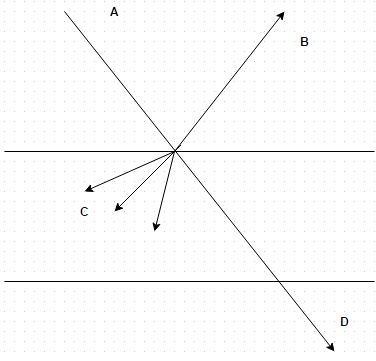Courses

# Test: Reflectivity

## 10 Questions MCQ Test Heat Transfer | Test: Reflectivity

Description
This mock test of Test: Reflectivity for Chemical Engineering helps you for every Chemical Engineering entrance exam. This contains 10 Multiple Choice Questions for Chemical Engineering Test: Reflectivity (mcq) to study with solutions a complete question bank. The solved questions answers in this Test: Reflectivity quiz give you a good mix of easy questions and tough questions. Chemical Engineering students definitely take this Test: Reflectivity exercise for a better result in the exam. You can find other Test: Reflectivity extra questions, long questions & short questions for Chemical Engineering on EduRev as well by searching above.
QUESTION: 1

### Reflectivity is defined as

Solution:

It is total energy reflected by the body. Its value depends upon the nature of the surface of the body, its temperature and wavelength of incident radiations.

QUESTION: 2

### Of the radiant energy 350W/m2 incident upon a surface 250W/m2 is absorbed, 60W/m2 is reflected and the remainder is transmitted through the surface. Workout the value for reflectivity for the surface material

Solution:

Reflectivity = fraction of total energy reflected by the body = 60/350 = 0.171.

QUESTION: 3

### A thin metal plate of 4 cm diameter is suspended in atmospheric air whose temperature is 290 K. This plate attains a temperature of 295 K when one of its face receives radiant energy from a heat source at the rate of 2 W. If heat transfer coefficient on both surfaces of the plate is stated to be 87.5 W/m 2 K, workout the energy lost by reflection

Solution:

Heat loss by convection from both sides of the plates = 2 h A d t = 1.1 W. Energy lost by reflection = 2.0 – 1.1 = 0.9 W.

QUESTION: 4

The value of reflectivity depends upon
(i) Body temperature
(iii) Nature of surface of body
Identify the correct statement

Solution:

It should be wavelength of incident radiation. It is the fraction of total energy reflected by the body.

QUESTION: 5

When a surface absorbs a certain fixed percentage of striking radiation the surface is called

Solution:

It is absorbing a certain fixed amount of radiations.

QUESTION: 6

Which one is true regarding gases?

Solution:

Gases are known to reflect very little of the radiation incident on their surface.

QUESTION: 7

Thin glass plate is an example of

Solution:

For transparent body, transmissivity is zero.

QUESTION: 8

Radiant energy with an intensity of 800 W/m2 strikes a flat plate normally. The absorptivity is thrice the reflectivity and twice the transmissivity. Determine the rate of reflection

Solution:

Rate of reflection = pQ= 218.20 W/m2.

QUESTION: 9

The total radiant energy A impinging upon a body would be partially or totally absorbed, reflected from its surface or transmitted through it. Identify the correct statementSolution:

A = B + C + D.

QUESTION: 10

Which one is true for opaque body?

Solution:

For opaque body, sum of absorptivity and reflectivity is unity.

• Test